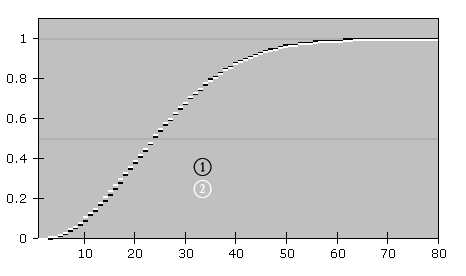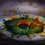# The Birthday problem... amazing !!!According to the "$$\color{Blue}{ \text{Pigeonhole}- \text{principle}}$$ " we know that if in a given set of people , you want to make sure there are 2 people with $$\color{Blue}{same}$$ $$\color{Blue}{ birthdays}$$ then you have to get 367 people in the set. (There are 366 choices for a birthday , therefore)

But do you know that $\color{#D61F06}{50}\%$ % probability of 2 people having same birthdays is actually achieved even in a set of 23 people !!!!!

And what's even more exciting is , $\color{#D61F06}{99.9 }\%$% probability is for the set of 70 people !!!!!

So hard to believe, but I have a mathematical function to show this, I saw it on the Wikipedia Page

Here, the probability of people having same birthday will be given by

$\color{#20A900}{p(n)}$ .. (probability in set of $n$ people) = $\color{#20A900}{1\times \biggl(1-\dfrac{1}{365}\biggr) \times \biggl(1-\dfrac{2}{365}\biggr) \times \biggl(1-\dfrac{3}{365}\biggr)\times .... \times \biggl(1-\dfrac{n}{365}\biggr)}$

That is $\color{#69047E}{p(n) = \dfrac{365!}{365^n \times (365-n)! }= \dfrac{n! \times \dbinom{365}{n}}{365^n}}$

The graph of probability (of course it won't be a continuous graph because number of people has to be an integer) is as shown in attached image.

This shows how rapidly the graph grows in first few numbers.....

To know more about this problem, go to the wikipedia page ....Note by Aditya Raut
7 years ago

This discussion board is a place to discuss our Daily Challenges and the math and science related to those challenges. Explanations are more than just a solution — they should explain the steps and thinking strategies that you used to obtain the solution. Comments should further the discussion of math and science.

When posting on Brilliant:

• Use the emojis to react to an explanation, whether you're congratulating a job well done , or just really confused .
• Ask specific questions about the challenge or the steps in somebody's explanation. Well-posed questions can add a lot to the discussion, but posting "I don't understand!" doesn't help anyone.
• Try to contribute something new to the discussion, whether it is an extension, generalization or other idea related to the challenge.
• Stay on topic — we're all here to learn more about math and science, not to hear about your favorite get-rich-quick scheme or current world events.

MarkdownAppears as
*italics* or _italics_ italics
**bold** or __bold__ bold
- bulleted- list
• bulleted
• list
1. numbered2. list
1. numbered
2. list
Note: you must add a full line of space before and after lists for them to show up correctly
paragraph 1paragraph 2

paragraph 1

paragraph 2

[example link](https://brilliant.org)example link
> This is a quote
This is a quote
    # I indented these lines
# 4 spaces, and now they show
# up as a code block.

print "hello world"
# I indented these lines
# 4 spaces, and now they show
# up as a code block.

print "hello world"
MathAppears as
Remember to wrap math in $$ ... $$ or $ ... $ to ensure proper formatting.
2 \times 3 $2 \times 3$
2^{34} $2^{34}$
a_{i-1} $a_{i-1}$
\frac{2}{3} $\frac{2}{3}$
\sqrt{2} $\sqrt{2}$
\sum_{i=1}^3 $\sum_{i=1}^3$
\sin \theta $\sin \theta$
\boxed{123} $\boxed{123}$

## Comments

Sort by:

Top Newest

Actually if you think about it and calculate the numbers you can get this easily... it isn't rocket science.

- 7 years ago

Log in to reply

But it is really not felt at the beginning that 50% probability will be achieved by 6% of the population needed to assure 100% probability !!!! @Joshua Ong .... that's why i felt like sharing here....it's very easy yet totally never tried thing, many people might have never tried to know what number of people gives what probability.... nothing new technique... but unexpected results !

- 7 years ago

Log in to reply

×

Problem Loading...

Note Loading...

Set Loading...